Particle-in-cell (PIC) method is an effective simulation tool for plasma kinetics, where velocity distribution of plasma species deviates from equilibrium and often does not remain stationary. While mesh-based methods suffer from high-dimensional space-velocity phase space, PIC method utilizes coarse-grained Lagrangian particles to represent the plasma distribution with amenable computational costs.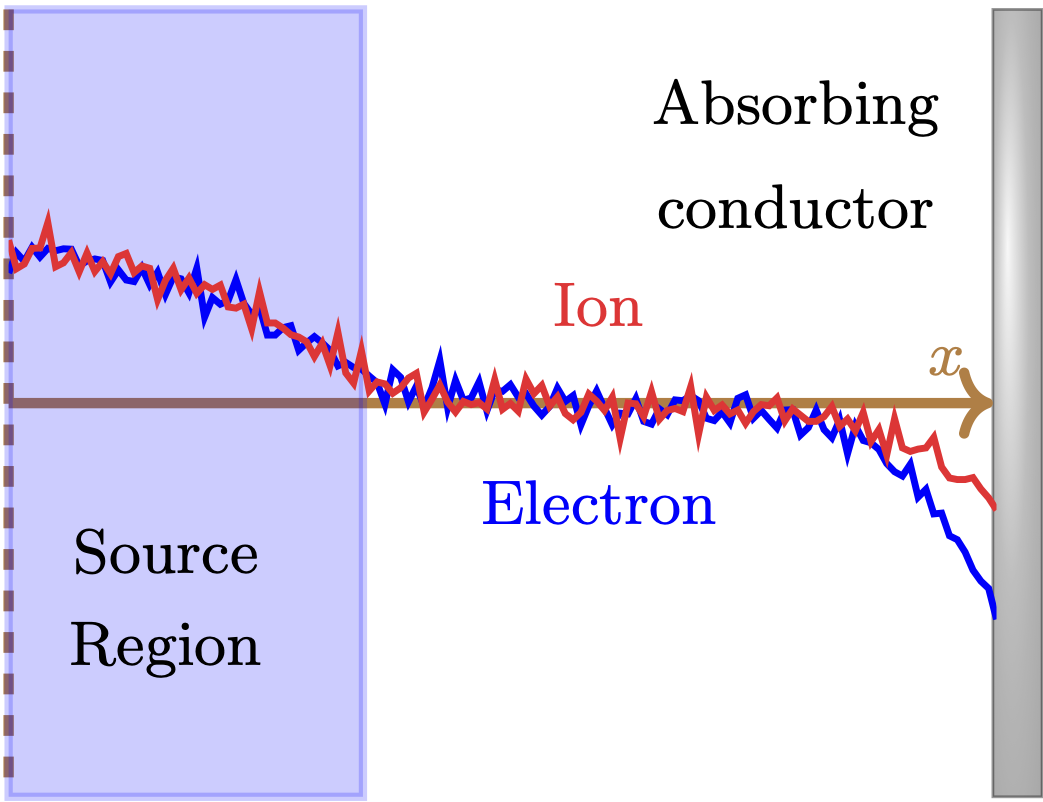PIC simulation of a sheath edge formation: number densities of electrons and ions (left); space-velocity distribution of electrons (top right) and ions (bottom right).

PIC simulation can benefit from sensitivity analysis, which provides a gradient of a quantity-of-interest $$\mathcal{J}$$ to parameters-of-interests $$\mathbf{\Theta}$$. Such gradient can be used to:

• design optimization
• uncertainty quantification.

Computing the sensitivity in PIC simulations, however, is challenging. Simulation particles mimicks the chaotic $$N$$-body dynamics of actual molecules, which are extremely sensitive to their initial conditions. While there is an ‘useful’ sensitivity of the plasma distribution as a whole, exploding sensitivities of individual particles mask it to be computed exactly.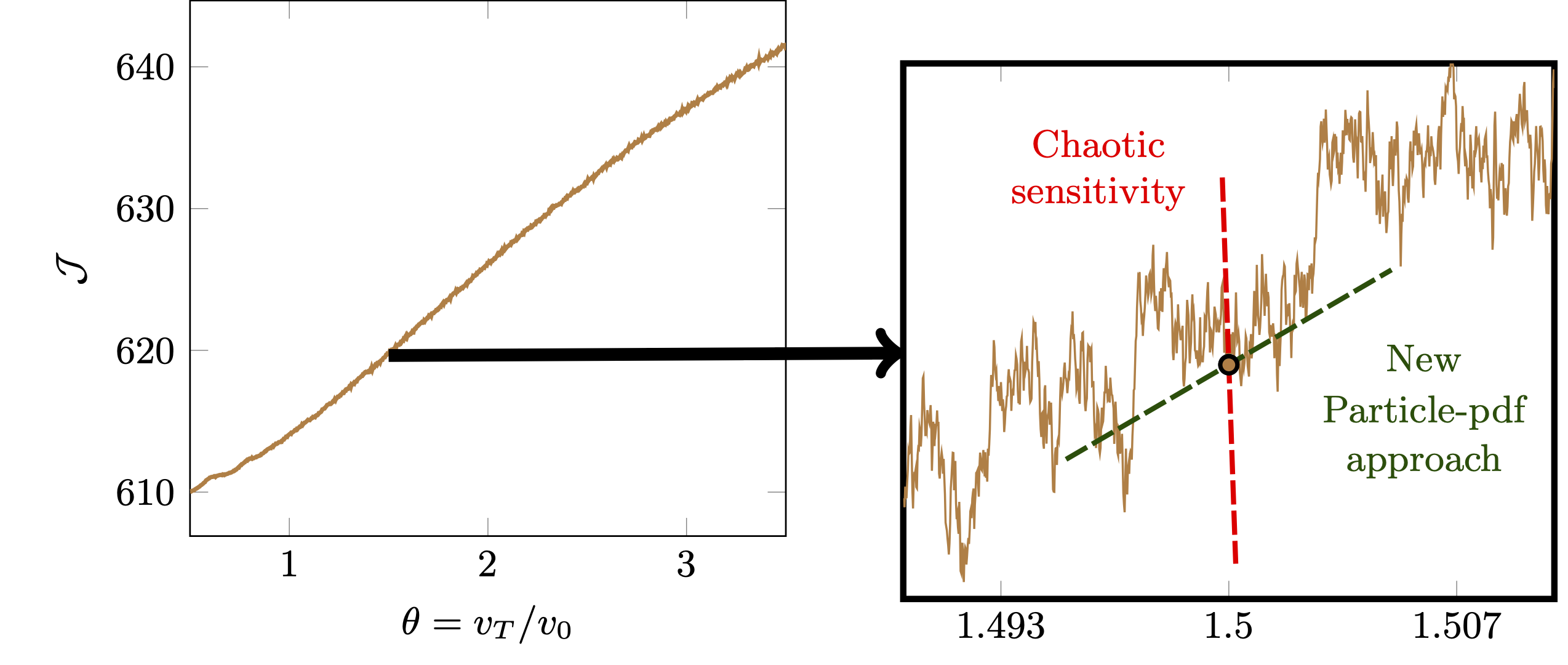Debye-shielding length scale $\mathcal{J}$ as a function of electron thermal velocity $v_T$ (left). Due to chaotic particle dynamics, $\mathcal{J}(v_T)$ becomes extremely non-smooth (right).

I proposed and developed the particle-pdf approach, where the adjoint particles represent the sensitivity of plasma distribution, not individual plasma particles. The proposed approach avoids chaotic sensitivities of particles and computes the useful sensitivity at a computational cost similar to the original plasma simulation.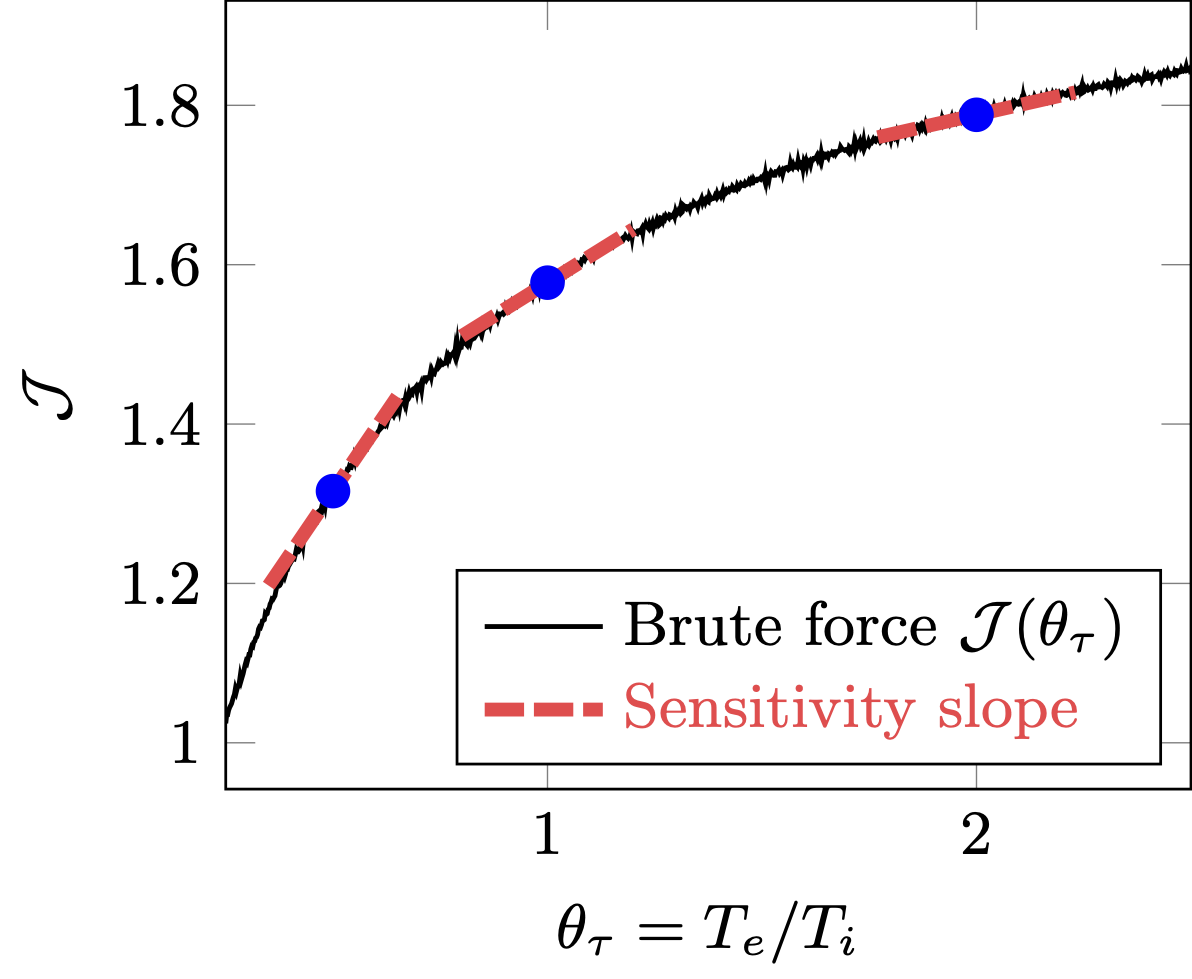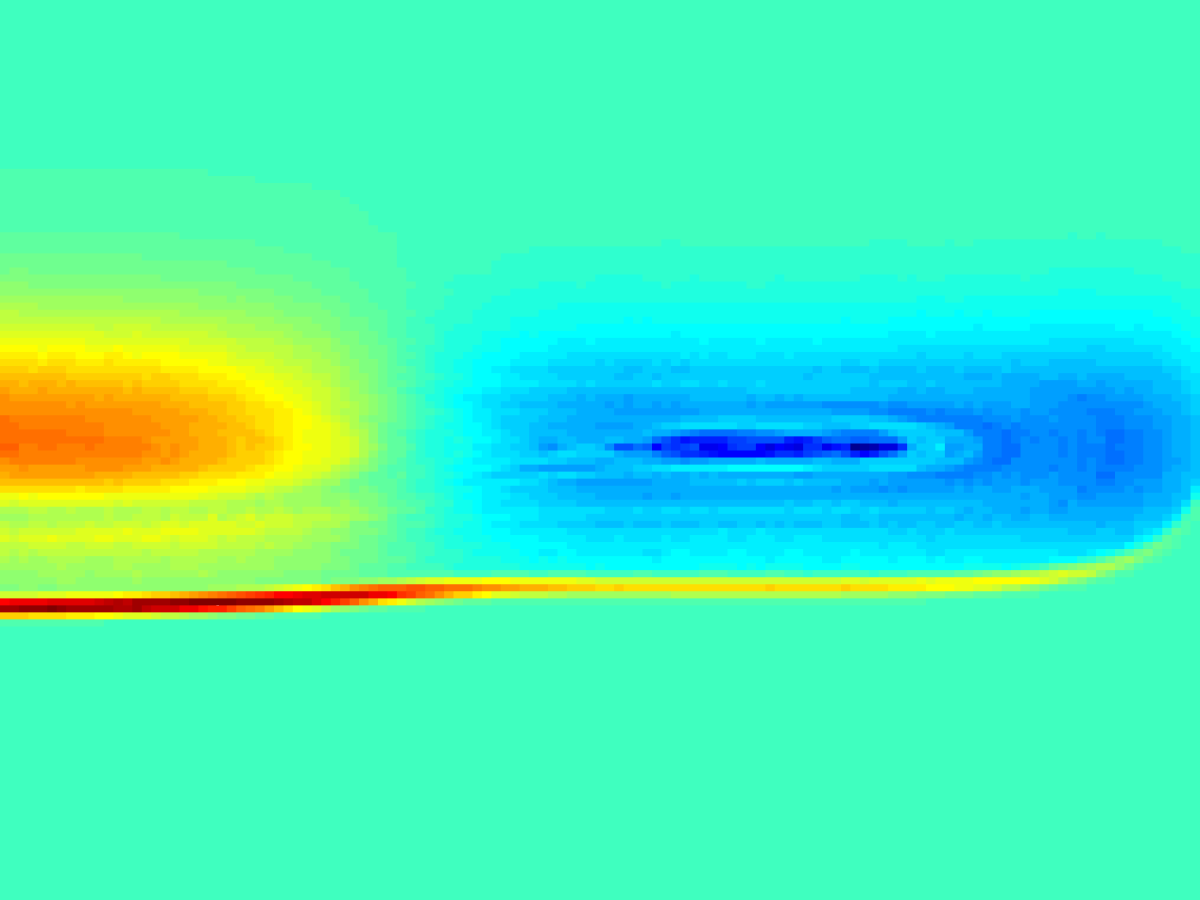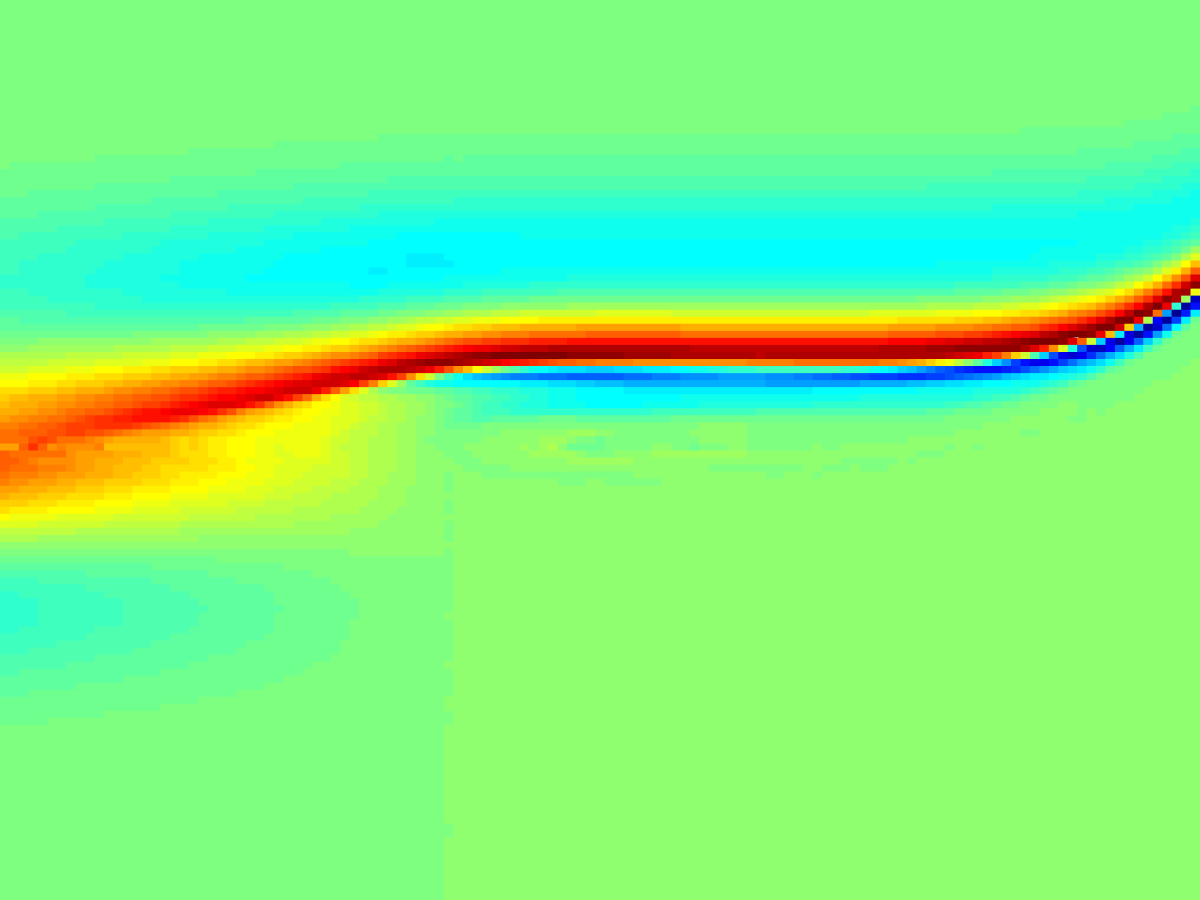Sensitivity of the potential drop to the temperature ratio (left), in the sheath-edge formation. Electron sensitivity distribution (top-right) and ion sensitivity distribution (bottom-right).